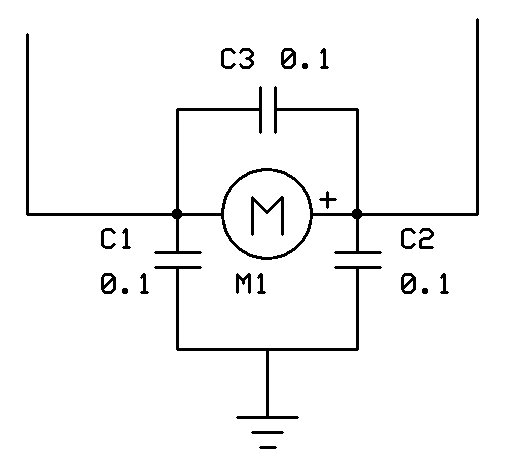# Possible noise from the motors

Hi Everyone,

I'm trying to control a self-balancing car, but it seems that the MPU6050 get dirty once my motors operate a full velocity.
I'm controlling my motors with the module L298N and with the PWM signal (pin 5, 6, 9 and 10 from the arduino).
I have read about decoupling it, with capacitors, but I don't know exactly how should I do it.

This is my code. It's a little messy (sorry for that, it just I'm still figuring out what is going on) and also an image of my project:

``````#include <PID_v1.h>
#include <LMotorController.h>
#include "I2Cdev.h"
#include "MPU6050_6Axis_MotionApps20.h"

#if I2CDEV_IMPLEMENTATION == I2CDEV_ARDUINO_WIRE
#include "Wire.h"
#endif

#define MIN_ABS_SPEED 20

MPU6050 mpu;

#define DEBUG
//#define OFFSET_1

//#define STOP

// MPU control/status vars
bool dmpReady = false; // set true if DMP init was successful
uint8_t mpuIntStatus; // holds actual interrupt status byte from MPU
uint8_t devStatus; // return status after each device operation (0 = success, !0 = error)
uint16_t packetSize; // expected DMP packet size (default is 42 bytes)
uint16_t fifoCount; // count of all bytes currently in FIFO
uint8_t fifoBuffer; // FIFO storage buffer

// orientation/motion vars
Quaternion q; // [w, x, y, z] quaternion container
VectorFloat gravity; // [x, y, z] gravity vector
float euler; // [psi, theta, phi]    Euler angle container

//PID
//double originalSetpoint = 175.8;
#ifdef OFFSET_1
double originalSetpoint = 225;
#else
double originalSetpoint = 184;
#endif

double setpoint = originalSetpoint;
double movingAngleOffset = 0.1;
double input, output, output_left, output_right, error;
int moveState=0; //0 = balance; 1 = back; 2 = forth
double Kp = 40;
double Kd = 0.05;
double Ki = 5;
PID pid(&input, &output, &setpoint, Kp, Ki, Kd, DIRECT);

double motorSpeedFactorLeft = 1;
double motorSpeedFactorRight = 1;
double motorSpeedFactorForward = 1;
double motorSpeedFactorBackward = 1;

//MOTOR CONTROLLER
int ENA = 5;
int IN1 = 6;
int IN2 = 7;
int IN3 = 8;
int IN4 = 9;
int ENB = 10;
//LMotorController motorController(ENA, IN1, IN2, ENB, IN3, IN4, motorSpeedFactorLeft, motorSpeedFactorRight);

//timers
long time1Hz = 0;
long time5Hz = 0;

volatile bool mpuInterrupt = false; // indicates whether MPU interrupt pin has gone high
{
mpuInterrupt = true;
}

void setup()
{

// join I2C bus (I2Cdev library doesn't do this automatically)
#if I2CDEV_IMPLEMENTATION == I2CDEV_ARDUINO_WIRE
Wire.begin();
Wire.setClock(400000);  // Set the SCL clock speed to 400kHz
//Wire.setClock(200000);  // Set the SCL clock speed to 400kHz
//TWBR = 24; // 400kHz I2C clock (200kHz if CPU is 8MHz)
#elif I2CDEV_IMPLEMENTATION == I2CDEV_BUILTIN_FASTWIRE
Fastwire::setup(400, true);
//Fastwire::setup(200, true);
#endif

Serial.begin(115200);
mpu.initialize();

devStatus = mpu.dmpInitialize();

// supply your own gyro offsets here, scaled for min sensitivity

#ifdef OFFSET_1
mpu.setXGyroOffset(0);
mpu.setYGyroOffset(0);
mpu.setZGyroOffset(0);
mpu.setZAccelOffset(16372);
mpu.setYAccelOffset(5);
mpu.setXAccelOffset(2);
#else
mpu.setXGyroOffset(-52);
mpu.setYGyroOffset(-12);
mpu.setZGyroOffset(53);
mpu.setZAccelOffset(2420);
mpu.setYAccelOffset(2881);
mpu.setXAccelOffset(-784);
#endif

// make sure it worked (returns 0 if so)
if (devStatus == 0)
{

//mpu.CalibrateAccel(6);
//mpu.CalibrateGyro(6);
// turn on the DMP, now that it's ready
mpu.setDMPEnabled(true);

// enable Arduino interrupt detection
mpuIntStatus = mpu.getIntStatus();

// set our DMP Ready flag so the main loop() function knows it's okay to use it

// get expected DMP packet size for later comparison
packetSize = mpu.dmpGetFIFOPacketSize();

pid.SetMode(AUTOMATIC);
pid.SetSampleTime(10);
pid.SetOutputLimits(-255, 255);
}
else
{
// ERROR!
// 1 = initial memory load failed
// 2 = DMP configuration updates failed
// (if it's going to break, usually the code will be 1)
Serial.print(F("DMP Initialization failed (code "));
Serial.print(devStatus);
Serial.println(F(")"));
}

pinMode(11, OUTPUT);
pinMode(12, OUTPUT);

pinMode(5, OUTPUT);
pinMode(6, OUTPUT);

pinMode(9, OUTPUT);
pinMode(10, OUTPUT);

}
void loop()
{
#ifdef STOP
return;
#endif

// if programming failed, don't try to do anything

// wait for MPU interrupt or extra packet(s) available
while (!mpuInterrupt && fifoCount < packetSize)
{
pid.Compute();

output = 255;
output_left = output * motorSpeedFactorLeft;
output_right = output * motorSpeedFactorRight;
if(input > (setpoint))
{
output_left = output_left*motorSpeedFactorForward ;
output_right = output_right* motorSpeedFactorForward;

Move_Forward();
}
else if(input < (setpoint))
{
output_left = output_left*motorSpeedFactorBackward ;
output_right = output_right* motorSpeedFactorBackward;
Move_Backward();
}
else
{
Stop();
}
}
// reset interrupt flag and get INT_STATUS byte
mpuInterrupt = false;
mpuIntStatus = mpu.getIntStatus();

// get current FIFO count
fifoCount = mpu.getFIFOCount();

// check for overflow (this should never happen unless our code is too inefficient)
if ((mpuIntStatus & 0x10) || fifoCount == 1024)
{
// reset so we can continue cleanly
mpu.resetFIFO();
Serial.println(F("FIFO overflow!"));

// otherwise, check for DMP data ready interrupt (this should happen frequently)
}
else if (mpuIntStatus & 0x02)
{
// wait for correct available data length, should be a VERY short wait
while (fifoCount < packetSize) fifoCount = mpu.getFIFOCount();

// read a packet from FIFO
mpu.getFIFOBytes(fifoBuffer, packetSize);

// track FIFO count here in case there is > 1 packet available
// (this lets us immediately read more without waiting for an interrupt)
fifoCount -= packetSize;

mpu.dmpGetQuaternion(&q, fifoBuffer);
mpu.dmpGetGravity(&gravity, &q);
mpu.dmpGetYawPitchRoll(euler, &q, &gravity);
input = (euler * 180 / M_PI + 180);
error = input - setpoint;
//    Serial.print("\tOutput: ");
//    Serial.print(output);
Serial.print("\tError: ");
Serial.println(error);

}
}

void Move_Forward() //Code to rotate the wheel forward
{

//******RIGHT******//
analogWrite(5, -1*output_right);
analogWrite(6, 0);

//******LEFT******//
analogWrite(9, -1*output_left);
analogWrite(10, 0);

digitalWrite(11, HIGH);
digitalWrite(12, LOW);
}

void Move_Backward() //Code to rotate the wheel forward
{
//******RIGHT******//
analogWrite(5, LOW);
analogWrite(6, 1*output_right);

//******LEFT******//
analogWrite(9, 0);
analogWrite(10, 1*output_left);

digitalWrite(11, LOW);
digitalWrite(12, HIGH);
}

void Stop() //Code to rotate the wheel forward
{
analogWrite(11, LOW);
analogWrite(12, LOW);
analogWrite(5, LOW);
analogWrite(6, LOW);

digitalWrite(9, LOW);
digitalWrite(10, LOW);
}
``````

OP image

How to post images so we don't have to download them and everyone can see them.
How to post an image.
Another page on posting images.

Here is how to connect capacitors to a motor to decouple motor noise.The L298 drivers are ancient and inefficient technology. They are ill suited for battery power projects as they waste a lot of power as heat. Pololu (and others) have modern, much more efficient drivers at low cost.